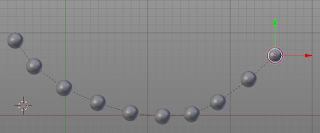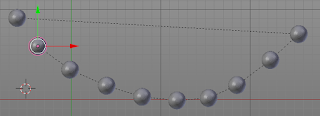### Add-on: Creating a chain of objects

While working on a project I came across a problem that is surprisingly hard to tackle: chaining a collection of objects along the shortest path.

What I want for this specific example is to parent a collection of objects to each other in such a way that we have a linear chain of parent-child relations. On top of that I want this chain to be as short as possible, that is, going from parent to child along the chain of objects, I want the length of this path to be minimal.

To illustrate what I mean, the first image shows what I am after while the second image shows a decidedly sub-optimal chain of parent-child relations:The problem itself is well known (finding the shortest Hamiltonian path, closely related to the Traveling Salesman problem) but unfortunately solving this problem exactly is very costly in computational terms.

The code below shows a working but very naive implementation that I intend to use as a starting point for later improvements. It works in the sense that it finds the shortest path between a collection of selected objects and creates the chain of parent-child relations but the time to compute the solution increases more that exponentially (factorial to be precise: 8 objects will for example take 0.1 seconds, 9 objects will take nine times as much, i.e. almost 1 second and 10 objects will take ten times as much still, i.e. 10 seconds and so on). To make this remotely useful, for example to chain a necklace of 100 beads, we will have to implement some clever heuristics. That is something I intend to cover in future articles.

## Code availability

The current code is shown in full below but the add-on as it evolves will be available on GitHub. (click 'Raw' to download the Python file)
```import bpy
from mathutils import kdtree
from itertools import permutations as perm
from functools import lru_cache
from time import time
from math import factorial as fac

bl_info = {
"name": "Chain selected objects",
"author": "Michel Anders (varkenvarken)",
"version": (0, 0, 201701220957),
"blender": (2, 78, 0),
"location": "View3D > Object > Chain selected objects",
"description": """Combine selected objects to a list of parent-child relations based on proximity""",
"category": "Object"}

def object_list(objects):
"""
Return the shortest Hamiltonian path through a collection of objects.

This is calculated using a brute force method that is certainly not
intented for real life use because for example going from ten to
eleven objects will increase the running time elevenfold and even
with caching expensive distance calculations this quickly becomes
completely unworkable.

But this routine is intended as our baseline algorithm that is meant
to be replaced with an approximation algorithm that is 'good enough'
for our purposes.
"""
@lru_cache()
def distance_squared(a,b):
return (objects[a].location-objects[b].location).length_squared

def length_squared(chain):
sum = 0.0
for i in range(len(chain)-1):
sum += distance_squared(chain[i],chain[i+1])
return sum

s = time()

shortest_d2 = 1e30
shortest_chain = None

n_half = fac(len(objects))//2
for i,chain in enumerate(perm(range(len(objects)))):
if i >= n_half:
break
d2 = length_squared(chain)
if d2 < shortest_d2:
shortest_d2 = d2
shortest_chain = chain

print("{n:d} objects {t:.1f}s".format(t=time()-s, n=len(objects)))

return [objects[i] for i in shortest_chain]

class ChainSelectedObjects(bpy.types.Operator):
bl_idname = 'object.chainselectedobjects'
bl_label = 'Chain selected objects'
bl_options = {'REGISTER', 'UNDO'}

@classmethod
def poll(self, context):
return (context.mode == 'OBJECT'
and len(context.selected_objects) > 1)

def execute(self, context):
objects = object_list(context.selected_objects.copy())
for ob in objects:
ob.select = False

ob = objects.pop()
first = ob
while objects:
context.scene.objects.active = ob
child = objects.pop()
child.select = True
bpy.ops.object.parent_set(keep_transform=True)
child.select = False
ob = child
first.select = True
context.scene.objects.active = first
return {"FINISHED"}

self.layout.operator(
ChainSelectedObjects.bl_idname,
text=ChainSelectedObjects.bl_label,
icon='PLUGIN')

def register():
bpy.utils.register_module(__name__)